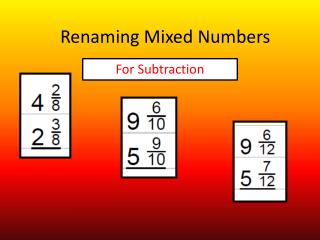DownloadDownload PresentationRenaming Mixed Numbers

# Renaming Mixed Numbers

Télécharger la présentation## Renaming Mixed Numbers

- - - - - - - - - - - - - - - - - - - - - - - - - - - E N D - - - - - - - - - - - - - - - - - - - - - - - - - - -
##### Presentation Transcript

1. Renaming Mixed Numbers For Subtraction

2. All the numerators in the first fraction are too small to subtract the second numerator. We have to change the way these mixed numbers look without changing their value. Renaming Mixed Numbers For Subtraction

3. FIRST-You have to find common denominators! Renaming Mixed Numbers For Subtraction

4. Renaming Mixed Numbers Renaming Mixed Numbers Let’s take it STEP BY STEP PRACTICE

5. Renaming the mixed number does not change its value. All three sections of this chart show the same value. Where is whole number 4 in this middle section? HINT: When the numerator and denominator are the same ~ the fraction is equal to 1.

6. Renaming the mixed number does not change its value. All three sections of this chart show the same value. Where does the improper fraction in this section come from? HINT: The two fractions are put together to make a fraction with a larger numerator so that subtraction will be possible.